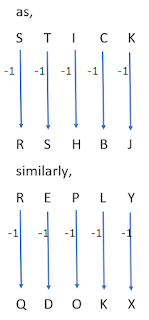# if STICK is coded as RSHBJ then REPLY is coded as

Coding Decoding Question is:

if STICK is coded as RSHBJ then REPLY is coded as

QDOKX

Detailed Solution is:

Let A=1, B=2, C=3,……. Z=26

As,

S-1=R

T-1=S

I-1=H

C-1=B

K-1=J

Similarly,

R-1=Q

E-1=D

P-1=O

L-1=K

Y-1=X

Hence, REPLY is coded as QDOKX.

Venn Diagram Method: